# Multiply and Divide by Powers of Ten

Interestingly, this was one of the most difficult lessons we’ve taught this year, both for teachers and students. There are several possible reasons:

1. Students come to the lesson with memorized algorithmic rules that are not grounded in understanding. Examples:
• “Multiply by 10, add a zero at the end”. If we ask “Why?”, there is a shrug or a muddled answer. Plus, this rule doesn’t work with decimals.
• “Just move the decimal point to the right that many places… or is it to the left?” Again, students cannot explain why this works. Plus – why does the decimal point move? And why to the LEFT (which is the direction of BIGGER numbers) when you DIVIDE? And how many places? Why count the zeroes??? “You just have to remember it” students assure us.
2. Students might not have a strong understanding of place value in base 10. Can they put it into words? What happens when you put 22 ones in the ones column? 122 ones? James Tanton’s “Exploding Dots” are a fun introduction to the meaning behind base 10: https://www.explodingdots.org/g/little-panda-17

## THE DECIMAL POINT DOESN’T MOVE

Here’s how it’s introduced in our Singapore Math book:What’s happening here?  Math is ALWAYS grounded in the real world. We HAVE to be able to show any concept with blocks. So give it a try:

Lay out one block  in the ones place: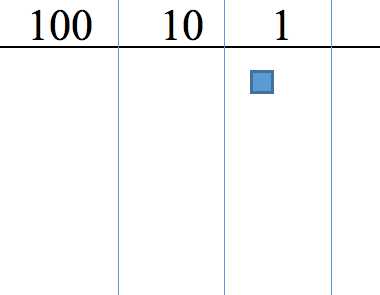Now multiply that “One” by 10. What happens? (It changes into a 10 rod, in the next column)  “Are you sure? How do you know?” (Because the rod equals 10 little “ones”. You can count them.)What happens if we multiply that TEN by 10?  (It turns into a 100-flat). “Are you sure? Can you prove it?” (The 100-flat is 10 times as big as a 10 rod — you can count the ten 10s in the 100-flat).Lets try it with numerals instead of blocks: Write the digits this time, and show 1 x 10.Why does the one move to the left? (multiplication makes things bigger) How many places? (one) Why? (times ten is the pattern across each of the base-10 place value headings in the chart, as you move to the left.)

And 1 times one hundred? (It moves TWO place value columns to the left) Why to the left (multiplication makes things bigger) How many places? (two) Why? (x 100 is times 10, times 10, or two columns on the base-10 place value headings in the chart)We didn’t start with blocks, because our students were exposed to them last year in fourth grade, but you certainly could.

We did start each of the first 2 days of this unit with a Power Point using place value.

## DAY ONE:

CWPowers10_1

Using these slides involves a lot of WAITING, so rally your patience!

Slide 1: Fraction Subtraction (Again!)  We are still having students draw fraction subtraction problems  (Like a vitamin — one a day!).  This ensures that our fastest, most algorithmic students learn to visualize what they otherwise see as ‘just numbers and steps’. It allows our most visual ‘builders’ to internalize the meaning of fraction subtraction before we approach the algorithm (which they would try to memorize but then confuse it with other algorithms like multiplication and division). By the time we get back to the algorithm (January?), they will have mastered the concept, and the algorithm will make sense.

Slide 2: Area Model puzzles (Again!)  We are still having students draw area models, with the “puzzle” component of finding a rectangle given its parts. In this case, (a) 1 flat, 6 rods, 8 cubes. (b) 1 flat, 7 rods, 12 cubes.  NOTE:  no multiplication necessary; you can simply count up the product. (100 + 6 tens + 8)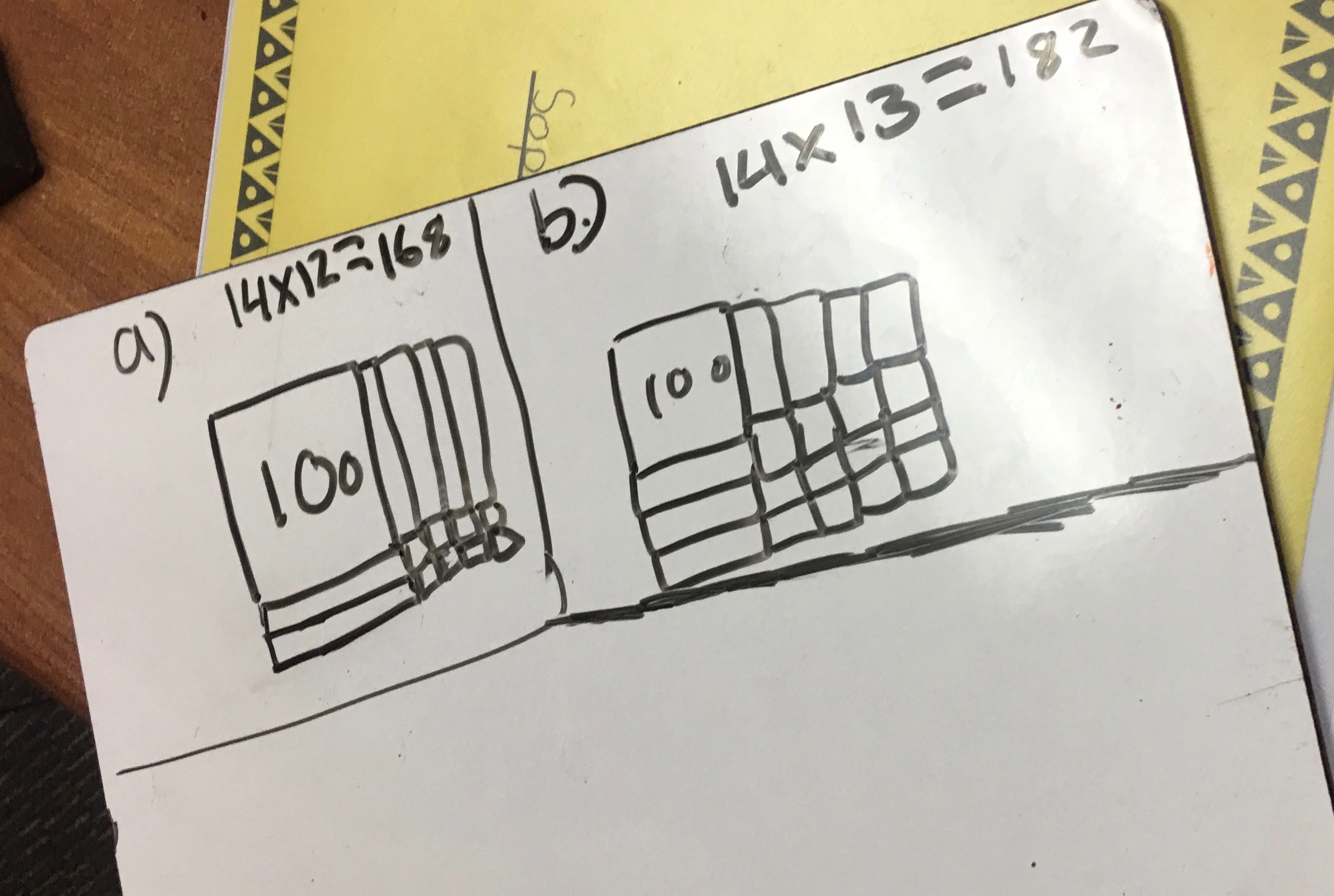Slide 4 & 5:  Students are working in pairs here. The only instruction is “WATCH what happens here. Can you figure it out? Explain what you see.” Be patient. Allow for various answers. The only goal is looking and thinking.

Rotation: This is optional, but rotating the pairs (Beta moves to the next Alpha) gives movement and allows for multiple interactions.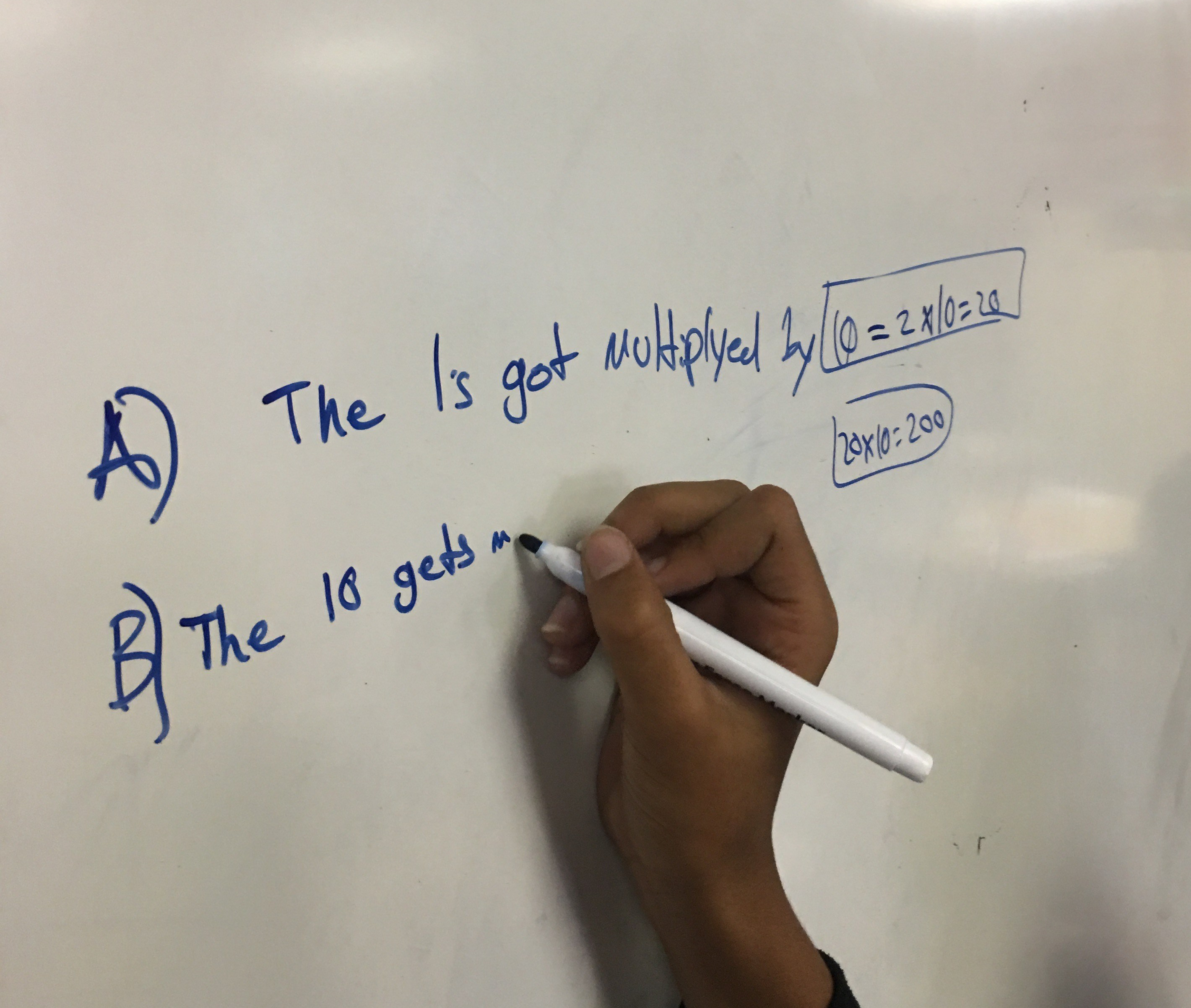NOTE: For part (b), a VERY common mistake is to multiply by 20, instead of 100. We just say “Hmmm… 2 x 20… can you show me with blocks?”  They quickly see the error. However, when we asked, almost NO one could tell us the difference between x 100 and x20. (one is 10 x 10, one is 10 + 10).  We let that go for now; a seed is planted.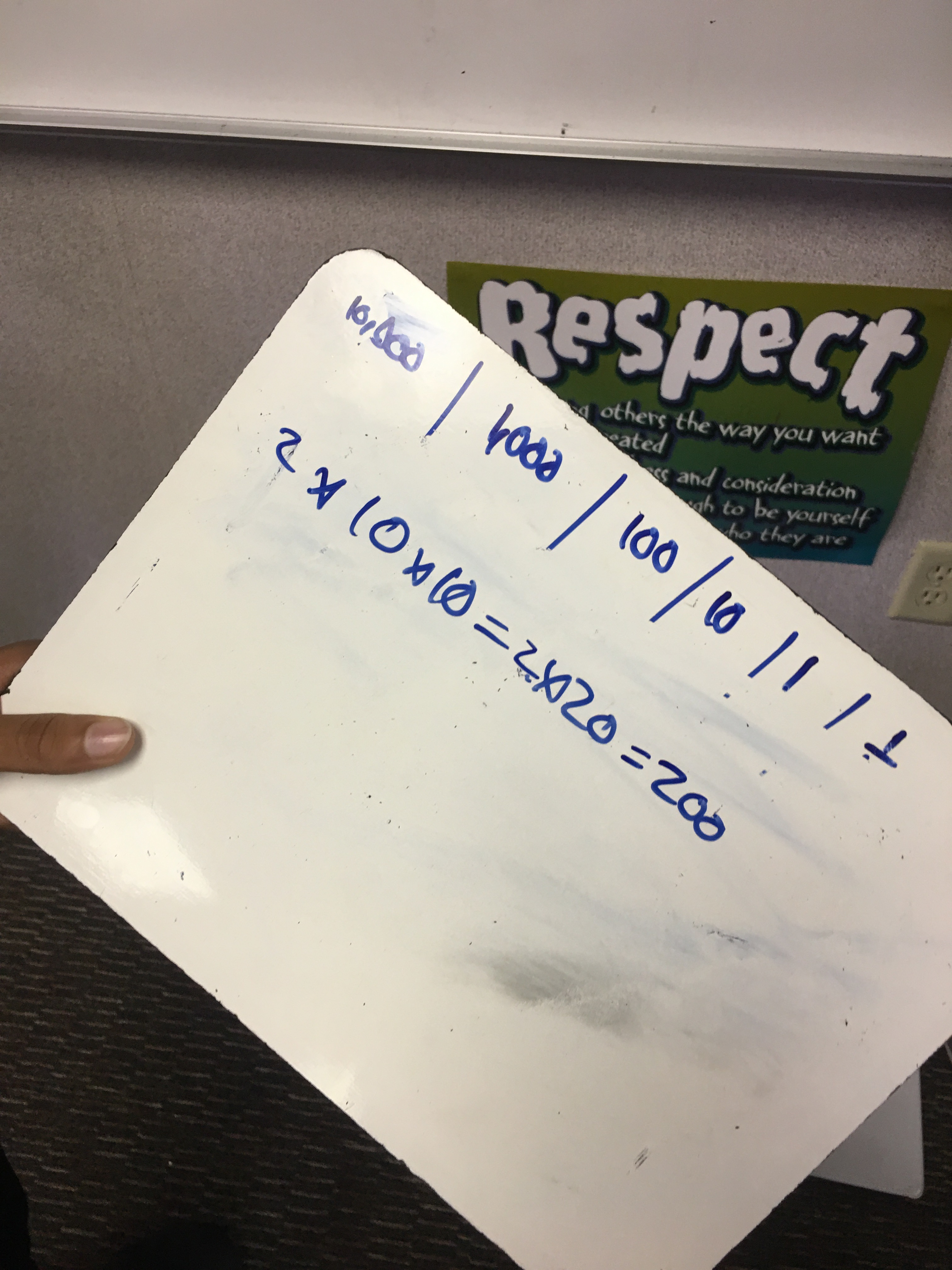Slides 7 & 8:  2 x 100 and 2 x 1000.Slide 9:  Write a “rule”.  This shows thoughts at the end of both days. (Mult and division. Feel free to break them apart)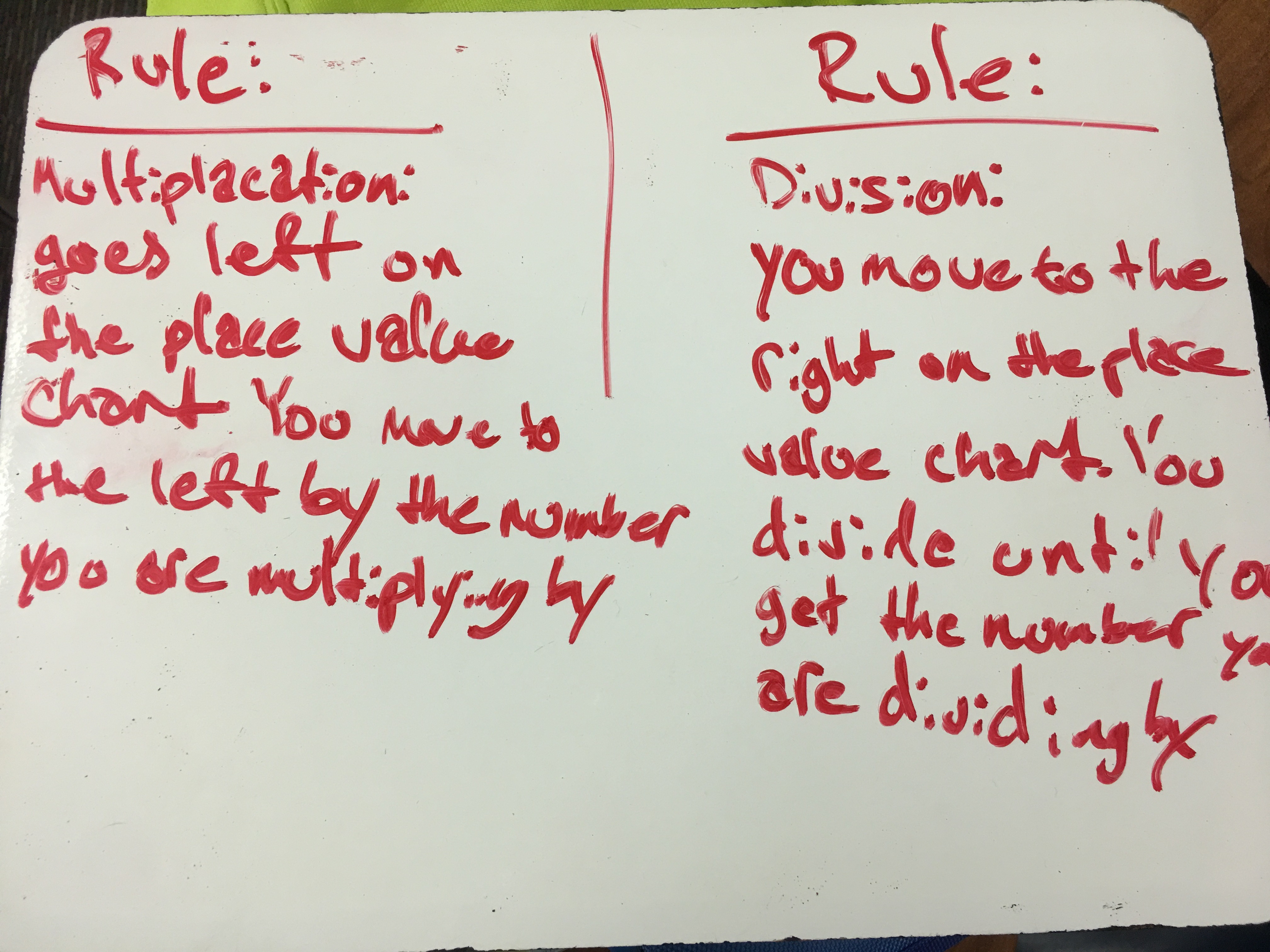Hmmm…  “I’m not sure what it means when it says “move to the left by the number you are multiplying by…?”

Confusion on “to the left?” … “To the right?” Below.Ahh… Now we’re getting there!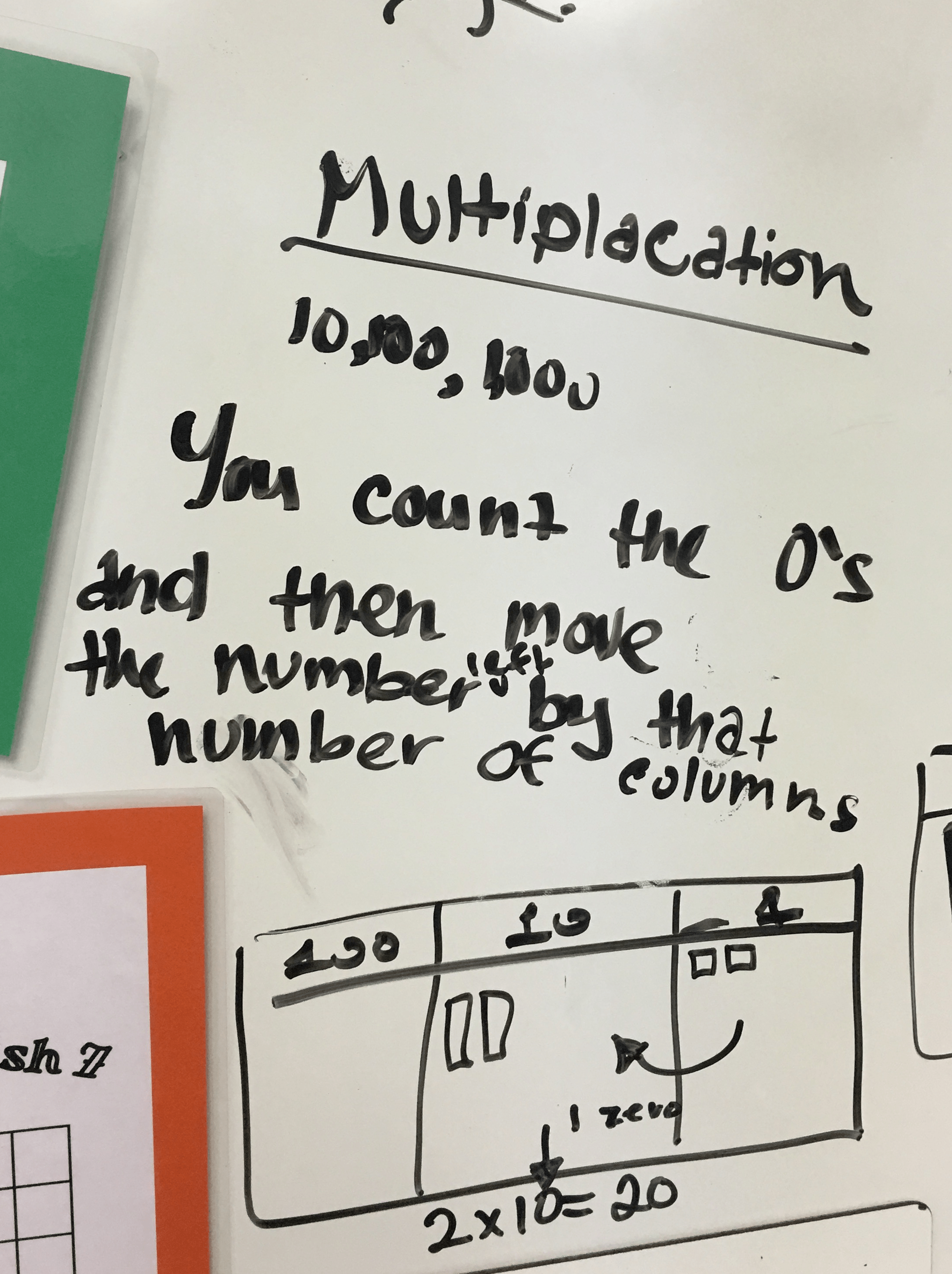## DAY ONE:

CWPowers10_2

This runs much as Day One. Give students TIME to put this into words and equations. At some point, you can point out that we can simply count the blocks at the end to find the final answer.

More coming soon!